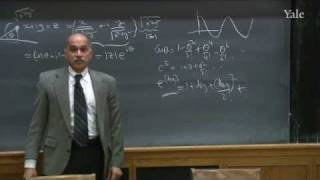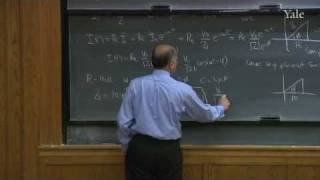﻿ Physics Fundamentals II Videos

# Physics Fundamentals II

• ### 11. Lenz's And Faraday's Laws

Fundamentals of Physics, II (PHYS 201) The electric effect of a changing magnetic field is described using Faraday's Law. The direction of the current so gen...

• ### 25. Quantum Mechanics VII: Summary Of Postulates And Special Topics

Fundamentals of Physics, II (PHYS 201) The various postulates of quantum mechanics treated in previous lectures are reviewed and summarized. The uncertainty ...

• ### 16. Ray Or Geometrical Optics I

Fundamentals of Physics, II (PHYS 201) Geometric optics is discussed as an approximation to wave theory when the wavelength is very small compared to other l...

• 1:09:57 Popular### 12. LCR Circuits—DC Voltage

Fundamentals of Physics, II (PHYS 201) Like capacitors, inductors act as energy storage devices in circuits. The relationship between voltage, inductance and...

• ### 23. Quantum Mechanics V: Particle In A Box

Fundamentals of Physics, II (PHYS 201) The allowed energy states of a free particle on a ring and a particle in a box are revisited. A scattering problem is ...

• ### 21. Quantum Mechanics III

Fundamentals of Physics, II (PHYS 201) The fact that the wave function provides the complete description of a particle's location and momentum is emphasized....

• ### 24. Quantum Mechanics VI: Time-dependent Schrödinger Equation

Fundamentals of Physics, II (PHYS 201) The time-dependent Schrödinger Equation is introduced as a powerful analog of Newton's second law of motion that descr...

• ### 4. Gauss's Law And Application To Conductors And Insulators

Fundamentals of Physics, II (PHYS 201) Lecture begins with a recap of Gauss's Law, its derivation, its limitation and its applications in deriving the electr...

• ### 18. Wave Theory Of Light

Fundamentals of Physics, II (PHYS 201) Young's double slit experiment shows clearly that light is a wave. (In order to observe the wave behavior of light, th...

• ### 6. Capacitors

Fundamentals of Physics, II (PHYS 201) The electric potential is defined for the electric field. It is introduced as an integral of the electric field making...

• ### 10. Ampere's Law

Fundamentals of Physics, II (PHYS 201) Ampere's Law is used to find the magnetic field generated by currents in highly symmetric geometries like the infinite...

• ### 17. Ray Or Geometrical Optics II

Fundamentals of Physics, II (PHYS 201) Ray diagrams are used to investigate the behavior of light incident on mirrors and lenses. The principle of least time...

• ### 3. Gauss's Law I

Fundamentals of Physics, II (PHYS 201) The electric field is discussed in greater detail and field due an infinite line charge is computed. The concepts of c...

• 1:10:52 Popular### 13. LCR Circuits—AC Voltage

Fundamentals of Physics, II (PHYS 201) The mathematics underlying LCR circuit theory for AC currents is discussed. Complex numbers are used to convert differ...

• ### 7. Resistance

Fundamentals of Physics, II (PHYS 201) Lecture begins with a discussion of electric potential distribution in conductors. Image charges are introduced and ex...

• ### 8. Circuits And Magnetism I

Fundamentals of Physics, II (PHYS 201) After a description of more complicated electric circuits, the basic ideas underlying magnetism are discussed and the ...

• ### 5. The Electric Potential And Conservation Of Energy

Fundamentals of Physics, II (PHYS 201) The law of conservation of energy is reviewed using examples drawn from Newtonian mechanics. The work-energy theorem i...

• ### 15. Maxwell's Equations And Electromagnetic Waves II

Fundamentals of Physics, II (PHYS 201) The physical meaning of the components of the wave equation and their applications are discussed. The power carried by...

• ### 19. Quantum Mechanics I: The Key Experiments And Wave-particle Duality

Fundamentals of Physics, II (PHYS 201) The double slit experiment, which implies the end of Newtonian Mechanics is described. The de Broglie relation between...

• ### 14. Maxwell's Equations And Electromagnetic Waves I

Fundamentals of Physics, II (PHYS 201) Waves on a string are reviewed and the general solution to the wave equation is described. Maxwell's equations in thei...

• ### 2. Electric Fields

Fundamentals of Physics, II (PHYS 201) The electric field is introduced as the mediator of electrostatic interactions: objects generate the field which perme...

• ### 9. Magnetism II

Fundamentals of Physics, II (PHYS 201) The mechanism by which electric currents produce a magnetic field (Law of Biot-Savart) is discussed in greater detail....